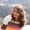FREELessons: 20Length: 1.7 hours• Overview
• Transcript

# 3.3 Ranges and the Double Dot Operator

In this lesson, I'll show you how ranges work in Kotlin. We'll look at ascending and descending ranges and the various range operators. I'll also show you how ranges are helpful when you're coding loops and iterators.

## 5.Conclusion1 lesson, 01:17

### 3.3 Ranges and the Double Dot Operator

Hello, in this lesson, we will be learning about ranges and double dot operators in Kotlin. This is going to be a very short lesson starting with the ranges. Let's say we have var range one, let me represent it as r1, is equal to 1..5. So here we have the variable r1, which has its range from 1 to 5. It will have the value, starting from 1 to 5. So this is the double dot operator, and the range of variable is 1, which ends at 5. Another way you can represent this range of r1 is, this is the range of r2. It is from 1 to 5. Same as the previous range, it is just a different representation. Now, what if we want the range in the descending order? It will be written like this. Say r3 =5..1 which will vary from 5, 4, 3, 2, 1. And now the other representation of this means 5 down to the other int value or we can say the to value is 1. Here instead of integer we can also have the characters. The value of this range will be varying from a, b, c, up to z. These ranges and double dot operators will be very helpful when we use it with the loop. Another way we can use these ranges and dot operators is if we want to have some steps in our ranges. Let's say if we want 1, 3, and 5, 1, 3, and 5. And we do not want 2 and 4. So how do we do that? 1..5 step 2. It means 1, the second one will be ignored, 3, fourth one will be ignored, and then 5. Which means at the step of 2, the values will be assigned to the ranges. Same in case of the down function. We will have var r7 = 5..1 step 2. Values will be 5, 3, 1. Same way instead of double dot operator you can use rangeTo to define the steps for your ranges. And similarly, for the descending order, you can use the downTo function and use the step with it. So here we are, we are done with the ranges and the double dot operators. In the next lesson, we will be learning about implementing these ranges and the double dot operators with the help of loops. So stay tuned for the upcoming videos. I hope you're having a great time. Keep smiling, and have a good day.

Back to the top